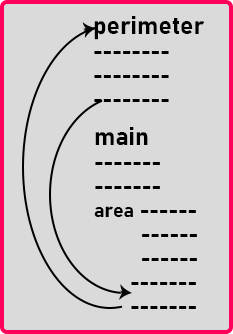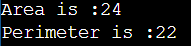# Scope Resolution Operator in C++

## Scope Resolution Operator in C++ with Examples:

In this article, I am going to discuss Scope Resolution Operator in C++ with Examples. Please read our previous article where we discussed Deep Copy and Shallow Copy Constructors in C++ with Examples.

##### What is Scope Resolution Operator in C++?

The Scope Resolution Operator in C++ is used to reference the global variable or member function that is out of scope. So, we use the scope resolution operator to access the hidden variable or function of a program. The operator is represented as the double colon (::) symbol. For example, when the global and local variable or function has the same name in a program, and when we call the variable, by default it only accesses the inner or local variable without calling the global variable. In this way, it hides the global variable or function. To overcome this situation, we need to use the scope resolution operator to fetch a program’s hidden variable or function.

##### Uses of Scope Resolution Operator in C++
1. It is used to access the global variables or member functions of a program when there is a local variable or member function with the same name
2. It is used to define the member function outside of a class.
3. It is also used to access the static variable and static function of a class.
4. In the case of multiple Inheritance, it is used to override the function.
5. For namespace: If a class having the same name exists inside two namespaces, we can use the namespace name with the scope resolution operator to refer to that class without any conflicts
6. Refer to a class inside another class: If a class exists inside another class, we can use the nesting class to refer to the nested class using the scope resolution operator

Note: In this article, we will discuss how to define member function outside a class using Scope Resolution Operator and the rest of the use cases we will discuss in our upcoming articles.

##### Scope Resolution Operator in C++:

In this article, first, we will discuss what are methods, and how we can write down the functions inside a class that is the member functions. Actually, there are two ways of writing member functions inside a class in C++.

To make you understand these two methods, please have a look at the below Rectangle class. Here, the Rectangle class has two private data members i.e. length and breadth. The Rectangle class also has one parameterized constructor to initialize the length and breadth data members. But here, from the below class let us keep the focus on the two methods i.e. area and perimeter.

```class Rectangle
{
private:
int length;
public:
Rectangle (int l, int b){
length = l;
}
int area ()
{
}
int perimeter ();
};
```

The first function that we have written in class is the area function. The area function will return length * breadth. We have written the body of a function inside the class itself. So, this is one approach to defining a function or method in C++.

The second approach is we have declared the function inside the class i.e. perimeter. We have not written the body of a function. For the second function, we have just given the prototype which is the declaration of a function inside the Rectangle class. Then where is the body of that function? Outside the class. So, we will write,

int perimeter(){
}

This is the body of function perimeter that we have defined outside the Rectangle class. This looks like a different function that is outside the class. Actually, we wanted to write down the body of the perimeter function that we declared inside the class.

Then we should tell the compiler that this perimeter function is the same as the perimeter function which was declared inside the class. So, for that we have to write the class name before the function name using the scope resolution operator (::) as shown below.

int Rectangle::perimeter(){
}

And after the class name and before the function name, we need to write the double colon that is scope resolution operator “::”. The scope resolution operator tells the scope of this function is within this class that is the function is already declared in that class.

So, we have two ways of writing a function inside a class. One is we need to elaborate the function inside the class itself by providing the function body. The second approach is just we need to write the prototype of the function inside the class and write down the function body outside the class using the scope resolution operator.

##### What are the differences between these two methods in C++?

Now let us see the difference between these two methods. So let us write the main function as follows:

int main(){
Rectangle r(8, 3);
cout << r.area();
cout << r.perimeter();
}

Here we have created a Rectangle object of length 8 and breadth 3 with the help of parameterized constructor. Next, we are printing the area and perimeter of object r. We have written the body of area function inside the class and the body of perimeter function outside the class using scoping resolution operator. When we compile this program, then this code will be converted into machine code and the machine code will look like the below.Let us assume that the dotted lines represent the machine instructions. Inside the main function, the object is created. Then the area will be called inside the main function. So, the machine code of the area is written here. The perimeter function will also be called inside the main function. You can see the machine code of the perimeter is outside the main function i.e. above the main function.

So, if we write the function outside the class using the scope resolution operator then the machine code for that function will be separately generated and when there is a call it will go to that function and after the function end, it will return back to the main function. If you are writing the function inside the class itself, then the machine code of that function will be replaced at the place of the function call.

So, in our example, the area function is not a separate function. The machine instructions of the area function are part of the main function only. So, it is a part of the main, it is not a separate area function.

Function means a separate piece of code must be there. For example, taking your clothes to the laundry and getting them washed. You have to go out from home and give clothes to the laundry and then they will wash and return your clothes. So, this is like a function.

But buying a washing machine and then washing the clothes at home. So, this area function is just like a washing machine that is laundry at home.

So, if the complete machine code is written here then it is not our function. It is a part of the main function. If you write down the functions inside the class only then such functions are called inline functions. These functions automatically become inline. Their machine code will be replaced wherever the function is called. In our next article, we will discuss Inline Functions in detail with Examples.

In C++, it will be a good programming practice to write the function outside the class using the scope resolution operator. Otherwise, if you write them inside the class then they will become inline functions. So, you should be careful when writing functions inside the class.

Inline functions should not have any complex logic. In our example, we are performing a simple arithmetic operation. But if there are loops and nested loops you should never write them inside the class. You should write their body outside the class. So that’s all about scope resolution operator in C++. Now let us write the complete program in C++ language.

##### Example to Understand Scope Resolution Operator in C++:
```#include <iostream>
using namespace std;
class Rectangle
{
private:
int length;
public:
Rectangle (int l, int b){
length = l;
}
int area ()
{
}
int perimeter ();
};
int Rectangle::perimeter(){